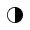• Resize textA -AA +
•# Seminar/ Colloquium

Home  »  Colloquium   »   From Calculus to Number Theory

## From Calculus to Number TheoryTitle : From Calculus to Number Theory.
Speaker : Prof. A. Raghuram, IISER, Pune.
Date : 13/02/2019, 5:30 PM , C.V Raman Hall, Second Floor.

Abstract:

I will start by recalling some classical formulae that one usually encounters in a first course in Calculus. For example, Euler proved in the 1730’s that the sum of reciprocals of squares of positive integers is one-sixth of the square of p. Such formulae are the prototypical examples of an entire of research in modern number theory called special values of L-functions. The idea of an L-function is crucial in the work of Andrew Wiles in his proof of Fermat’s Last Theorem. The aim of this lecture will be to give an appreciation for L-functions and to convey the grandeur of this subject that draws upon several different areas of mathematics such as representation theory, algebraic and differential geometry, and harmonic analysis. Towards the end of the talk, I will present some of my own recent results on the special values of certain automorphic L-functions.